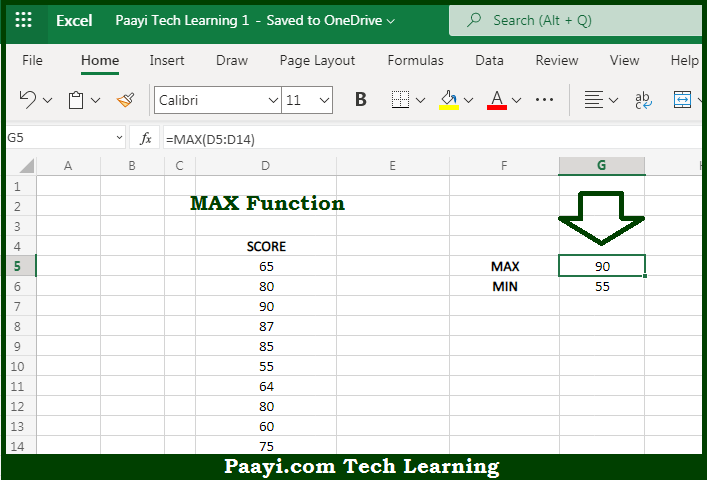# Learn How to Use Microsoft Excel MAX Function

Written by | 0 Comments | 539 Views

In this article, you will learn how to use the Microsoft Excel MAX function and its prime function in Microsoft Excel. You will also get to know the Microsoft Excel MAX function return value and syntax with the help of some examples.

Microsoft Excel MAX Function

The main purpose of the Microsoft Excel MAX function is to get the largest value. That implies, with the help of the MAX function you can able to return the largest numeric values based on their position in a list when sorted by value. It should be noted that The MAX function ignores empty cells, the logical values TRUE and FALSE, and text values. So, with the help of the MAX function, you can able to get the largest value in the provided values.

Return Value of MAX Function

The return value will be the largest value in the array.

Syntax of MAX Function

=MAX(number1, [number2], ...)

Where the arguments:

• number1: This is the Number, reference to a numeric value or range that contains numeric values.
• number2: This is the Number, reference to a numeric value or range that contains numeric values (optional).

## How to Use Microsoft Excel MAX Function?So we know that Microsoft Excel MAX function you can able to get the largest value. That implies, with the help of the MAX function you can able to return the largest numeric values based on their position in a list when sorted by value. It should be noted that The MAX function ignores empty cells, the logical values TRUE and FALSE, and text values. So, with the help of the MAX function, you can able to get the largest value in the provided values.